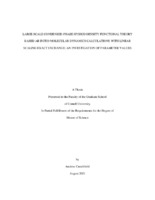## LARGE-SCALE CONDENSED-PHASE HYBRID DENSITY FUNCTIONAL THEORY BASED AB INITIO MOLECULAR DYNAMICS CALCULATIONS WITH LINEAR SCALING EXACT EXCHANGE: AN INVESTIGATION OF PARAMETER VALUES##### Abstract
Within computational chemistry inherent barriers exist when attempting to model real systems, and the primary barrier for modeling complex systems to a high degree of accuracy is the trade-off between numerical accuracy and computational expense. Large-scale condensed-phase hybrid density functional theory based ab initio molecular dynamics simulations attempt to accurately model real systems, but at high computational cost. Previous work in the DiStasio Group has produced methods to greatly increase the time efficiency of these intensive calculations, successfully reducing a calculation which typically undergoes cubic scaling with system size to a linear scaling regime. The purpose of this study is to investigate how the error of energy calculations changes by varying three parameters within the DiStasio Group’s exact exchange (EXX) program. These variables are c, a scaling factor for the dimensions of an orbital’s force domain, delta (δ), a filter for the amount of overlap required between two orbitals, and epsilon (ϵ), a tolerance factor for how well the bounding box of an orbital covers the orbital’s total electron density. Larger values for c ensure more accurate calculations since all force interactions are included in the energy calculations, but there is likely an optimal value which preserves desired numerical accuracy while reducing the computational expense of the calculation. When the sizes of δ and ϵ are too large the total energy of a system is undercalculated, so smaller values ensure a more complete energy profile. Though due to limitations in the Poisson solver which is implemented in the EXX program, additional issues with smooth calculation convergence exist when the variable δ is set below a certain threshold.
34 pages
2021-08
Zax, David B.
##### Committee Member
DiStasio, Jr, Robert A.
##### Degree Discipline
Chemistry and Chemical Biology
##### Degree Name
M.S., Chemistry and Chemical Biology
##### Degree Level
Master of Science
##### Types
dissertation or thesis